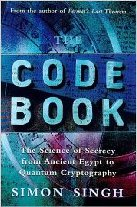# Cryptanalysis of the Affine Cipher

For a recap of how the affine cipher works, see here.

The affine cipher is very slightly more complicated than the Caesar cipher, but does not offer much more security. The number of possible keys is 12*26-1 = 311. This is very easy for a computer to simply search all possible keys and pick the best. To find the best key, we must try deciphering the ciphertext with each key, then determine the 'fitness' of each piece of deciphered text.

How do we determine how 'fit' a piece of text is? We calculate the statistics of the deciphered text, and compare these statistics to those calculated from standard english text. A fitness function will give us a number, the higher the number the more likely the particular key is the correct one. For this example we will be using quadgram statistics, but others are possible e.g. bigram or trigram statistics. These methods work by first determining the statistics of english text, then calculating the likelyhood that the ciphertext comes from the same distribution. An incorrectly deciphered (i.e. using the wrong key) message will probably contain sequences e.g. 'QKPC' which are very rare in normal english. In this way we can rank different decryption keys, the decryption key we want is the one that produces deciphered text with the fewest rare sequences.

The affine cipher has 2 key numbers, 'a' and 'b'. 'b' can range from 0 to 25, and 'a' can have any of the values 1,3,5,7,9,11,15,17,19,21,23,25. We iterate over each of these possible combinations, of which there are 311, determine the fitness of each combination, then chose the best.

## An Example §

Our ciphertext is the following:

```QUVNLAUVILZKVZZZVNHIVQUFSFZHWZQLQHQLJSNLAUVIFZLQ
LZYHKSVIFWKVQJFKKJMQUVFQQFNTZQUFQPJITFDFLSZQZHWZ
QLQHQLJSNLAUVIZLSFEELQLJSQJJQUVIFQQFNTZ```

We start decrypting with all possible keys (only a few are shown in the table):

``` a   b         plaintext             fitness
-----------------------------------------------
(1,   1)  PTUMKZTUHKYJUYYYUMGH... -1144.91
(3,   5)  VFOUCHFOBCYTOYYYOUSB... -1112.00
(11, 18)  OMFJXWMFSXDEFDDDFJZS... -1141.52
(17,  5)  THECIPHERISLESSSECUR...  -593.58
(21,  1)  XRWIYVRWJYQTWQQQWIEJ... -1194.72
(25,  9)  TPOWYJPOBYKZOKKKOWCB... -1117.56
```

As a result we find that the key corresponding to a=17, b=5 has the highest fitness, and indeed it is quite readable:

```THECIPHERISLESSSECURETHANASUBSTITUTIONCIPHERASIT
ISVULNERABLETOALLOFTHEATTACKSTHATWORKAGAINSTSUBS

## Python Code §

The code here uses pycipher for the Affine cipher. It implements the steps described above, using the ngram_score.py file available on the quadgram statistics page.The Code Book: The Science of Secrecy from Ancient Egypt to Quantum Cryptography ASIN/ISBN: 978-1857028799 Simon Singh's 'The Code Book' is an excellent introduction to ciphers and codes Buy from Amazon.com